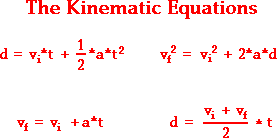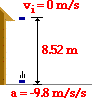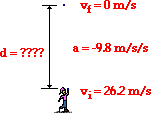1-D Kinematics - Lesson 6 - Describing Motion with Equations

# Kinematic Equations and Free FallAs mentioned in Lesson 5, a free-falling object is an object that is falling under the sole influence of gravity. That is to say that any object that is moving and being acted upon only be the force of gravity is said to be "in a state of free fall." Such an object will experience a downward acceleration of 9.8 m/s/s. Whether the object is falling downward or rising upward towards its peak, if it is under the sole influence of gravity, then its acceleration value is 9.8 m/s/s.

Like any moving object, the motion of an object in free fall can be described by four kinematic equations. The kinematic equations that describe any object's motion are:The symbols in the above equation have a specific meaning: the symbol d stands for the displacement; the symbol t stands for the time; the symbol a stands for the acceleration of the object; the symbol vi stands for the initial velocity value; and the symbol vf stands for the final velocity.

### Applying Free Fall Concepts to Problem-Solving

There are a few conceptual characteristics of free fall motion that will be of value when using the equations to analyze free fall motion. These concepts are described as follows:

• An object in free fall experiences an acceleration of -9.8 m/s/s. (The - sign indicates a downward acceleration.) Whether explicitly stated or not, the value of the acceleration in the kinematic equations is -9.8 m/s/s for any freely falling object.
• If an object is merely dropped (as opposed to being thrown) from an elevated height, then the initial velocity of the object is 0 m/s.
• If an object is projected upwards in a perfectly vertical direction, then it will slow down as it rises upward. The instant at which it reaches the peak of its trajectory, its velocity is 0 m/s. This value can be used as one of the motion parameters in the kinematic equations; for example, the final velocity (vf) after traveling to the peak would be assigned a value of 0 m/s.
• If an object is projected upwards in a perfectly vertical direction, then the velocity at which it is projected is equal in magnitude and opposite in sign to the velocity that it has when it returns to the same height. That is, a ball projected vertically with an upward velocity of +30 m/s will have a downward velocity of -30 m/s when it returns to the same height.

These four principles and the four kinematic equations can be combined to solve problems involving the motion of free falling objects. The two examples below illustrate application of free fall principles to kinematic problem-solving. In each example, the problem solving strategy that was introduced earlier in this lesson will be utilized.

### Example Problem A

Luke Autbeloe drops a pile of roof shingles from the top of a roof located 8.52 meters above the ground. Determine the time required for the shingles to reach the ground.

The solution to this problem begins by the construction of an informative diagram of the physical situation. This is shown below. The second step involves the identification and listing of known information in variable form. You might note that in the statement of the problem, there is only one piece of numerical information explicitly stated: 8.52 meters. The displacement (d) of the shingles is -8.52 m. (The - sign indicates that the displacement is downward). The remaining information must be extracted from the problem statement based upon your understanding of the above principles. For example, the vi value can be inferred to be 0 m/s since the shingles are dropped (released from rest; see note above). And the acceleration (a) of the shingles can be inferred to be -9.8 m/s2 since the shingles are free-falling (see note above). (Always pay careful attention to the + and - signs for the given quantities.) The next step of the solution involves the listing of the unknown (or desired) information in variable form. In this case, the problem requests information about the time of fall. So t is the unknown quantity. The results of the first three steps are shown in the table below.

Diagram: Given: Find:vi = 0.0 m/s
d = -8.52 m

a = - 9.8 m/s2
t = ??

The next step involves identifying a kinematic equation that allows you to determine the unknown quantity. There are four kinematic equations to choose from. In general, you will always choose the equation that contains the three known and the one unknown variable. In this specific case, the three known variables and the one unknown variable are d, vi, a, and t. Thus, you will look for an equation that has these four variables listed in it. An inspection of the four equations above reveals that the equation on the top left contains all four variables.

d = vi • t + ½ • a • t2

Once the equation is identified and written down, the next step involves substituting known values into the equation and using proper algebraic steps to solve for the unknown information. This step is shown below.

-8.52 m = (0 m/s) • (t) + ½ • (-9.8 m/s2) • (t)2

-8.52 m = (0 m) *(t) + (-4.9 m/s2) • (t)2

-8.52 m = (-4.9 m/s2) • (t)2

(-8.52 m)/(-4.9 m/s2) = t2

1.739 s2 = t2

t = 1.32 s

The solution above reveals that the shingles will fall for a time of 1.32 seconds before hitting the ground. (Note that this value is rounded to the third digit.)

The last step of the problem-solving strategy involves checking the answer to assure that it is both reasonable and accurate. The value seems reasonable enough. The shingles are falling a distance of approximately 10 yards (1 meter is pretty close to 1 yard); it seems that an answer between 1 and 2 seconds would be highly reasonable. The calculated time easily falls within this range of reasonability. Checking for accuracy involves substituting the calculated value back into the equation for time and insuring that the left side of the equation is equal to the right side of the equation. Indeed it is!

### Example Problem B

Rex Things throws his mother's crystal vase vertically upwards with an initial velocity of 26.2 m/s. Determine the height to which the vase will rise above its initial height.

Once more, the solution to this problem begins by the construction of an informative diagram of the physical situation. This is shown below. The second step involves the identification and listing of known information in variable form. You might note that in the statement of the problem, there is only one piece of numerical information explicitly stated: 26.2 m/s. The initial velocity (vi) of the vase is +26.2 m/s. (The + sign indicates that the initial velocity is an upwards velocity). The remaining information must be extracted from the problem statement based upon your understanding of the above principles. Note that the vf value can be inferred to be 0 m/s since the final state of the vase is the peak of its trajectory (see note above). The acceleration (a) of the vase is -9.8 m/s2 (see note above). The next step involves the listing of the unknown (or desired) information in variable form. In this case, the problem requests information about the displacement of the vase (the height to which it rises above its starting height). So d is the unknown information. The results of the first three steps are shown in the table below.

Diagram: Given: Find:vi = 26.2 m/s
vf = 0 m/s

a = -9.8 m/s2
d = ??

The next step involves identifying a kinematic equation that would allow you to determine the unknown quantity. There are four kinematic equations to choose from. Again, you will always search for an equation that contains the three known variables and the one unknown variable. In this specific case, the three known variables and the one unknown variable are vi, vf, a, and d. An inspection of the four equations above reveals that the equation on the top right contains all four variables.

vf2 = vi2 + 2 • a • d

Once the equation is identified and written down, the next step involves substituting known values into the equation and using proper algebraic steps to solve for the unknown information. This step is shown below.

(0 m/s)2 = (26.2 m/s)2 + 2 •(-9.8m/s2) •d

0 m2/s2 = 686.44 m2/s2 + (-19.6 m/s2) •d

(-19.6 m/s2) • d = 0 m2/s2 -686.44 m2/s2

(-19.6 m/s2) • d = -686.44 m2/s2

d = (-686.44 m2/s2)/ (-19.6 m/s2)

d = 35.0 m

The solution above reveals that the vase will travel upwards for a displacement of 35.0 meters before reaching its peak. (Note that this value is rounded to the third digit.)

The last step of the problem-solving strategy involves checking the answer to assure that it is both reasonable and accurate. The value seems reasonable enough. The vase is thrown with a speed of approximately 50 mi/hr (merely approximate 1 m/s to be equivalent to 2 mi/hr). Such a throw will never make it further than one football field in height (approximately 100 m), yet will surely make it past the 10-yard line (approximately 10 meters). The calculated answer certainly falls within this range of reasonability. Checking for accuracy involves substituting the calculated value back into the equation for displacement and insuring that the left side of the equation is equal to the right side of the equation. Indeed it is!

Kinematic equations provide a useful means of determining the value of an unknown motion parameter if three motion parameters are known. In the case of a free-fall motion, the acceleration is often known. And in many cases, another motion parameter can be inferred through a solid knowledge of some basic kinematic principles. The next part of Lesson 6 provides a wealth of practice problems with answers and solutions.

Next Section: